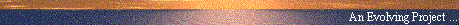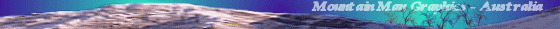# The Two Body Problem of General Relativity

#### by C. Hillman (sci.physics,sci.physics.relativity)

##### Web Publication by Mountain Man Graphics, Australia# The Two Body Problem of General Relativity

Date: Mon, 23 Nov 1998 16:13:21 -0800
From: "C. Hillman"
Newsgroups: sci.physics,sci.physics.relativity
Subject: Re: The Two Body Problem

```

>On 23 Nov 1998, Aaron Bergman wrote:
>> In article <36590BE3.12B6@magna.com.au>, Mountain Man wrote:

>>  What are the reasons for the hold-up in solving
>>  the 2-body problem?

> Well, er, GR is hard. Maybe Chris can give you a better answer
> than that.

I missed the original posting, but "GR is hard" is a good short answer.
More specifically, the field equation is nonlinear.

require a book length monograph which in fact someone probably ought to
write, someone like me, someone with really bad luck, since ;-) that would
increase the chances that the problem would be solved the day the
manuscript is sent to the printer; see for instance the summer school on
QFT which was "outdated" even as the course was in progress by the
preprint of Witten and Seiberg which had appeared that very week and which
very quickly led to the solution of all the outstanding problems the
course focused on, using methods superseded by the much simpler
Witten-Seiberg theory).

The best way to begin to understand why the two-body problem has proven so
hard, provided one has first mastered the basics of gtr from a book like
Wald or d'Inverno or Stephani or MTW or Huggett & Tod, is to get hold of
GRTensor or an equivalent package (see my relativity page; see url below)
and read my postings on solving the field equation by the (rather naive)
method of making a symmetry Ansatz.  In the simplest cases one can make an
Ansatz involving an isometry group with at least four parameters, which
can reduce the problem of solving the field equation to solving an
ordinary linear equation.

A particularly simple example (see my archived postings on my relativity
page for more detail) is given by the spherically symmetric static Ansatz

ds^2 = (f dt)^2 - (g r)^2 - r^2 ( du^2 + sin^2 u dv^2)

where f,g are functions of r only.  Computing the Einstein tensor in terms
of the unknown functions f,g and setting this equal to zero leads to quite
elementary ODES in terms of g,f which yield the Schwarzschild vacuum
solution

1/g = f = 1 - 2m/r

where m is a constant.  (The Einstein tensor of the original metric is
diagonal; demanding G^{tt} = G^{rr} gives g = 1/r and then putting these
equal to zero leads to f = 1 - 2m/r, which makes all four diagonal
components vanish.)  Finally, effective potential analysis of the
geodesics suggests and a weak-field analysis confirms that m may be
identified with the Keplerian mass.  Note that we used a geometrical
assumption to simplify the mathematical problem of solving the field
equation, and after solving the simplified problem still had to interpret
our solution in physical terms.  In many cases this last step is
considerably harder than this particular example suggests!

In the case of isolated rotating holes or axially symmetric collisions,
the maximal symmetry is axial symmetry and this turns out to lead to
equations sufficiently complex to resist solution by elementary means.
(Try it!)  But there is reason for hope--- in the case of isolated
rotating holes (which was an open problem for a long, long time) a
radically different idea leads to a simple trick from which the desired
Kerr solution drops right out.  (See for instance d'Inverno's textbook.)
It is quite possible, I guess, that a similar clever trick (which might
even occur to a math student relatively unfamiliar with the underlying
physics) might result in the first exact solution (four dimensional
spacetime) of the two body problem in gtr.  (Remark: there are preprints
on the Los Alamos server on n-body solutions in 2 dimensional gravity---
Steve Carlip could say much more than I could about how this work relates
to the four dimensional two body problem.)

Notice that by "two-body solution" we mean an exact vacuum solution which
can be shown to model two massive bodies (which could be stars or black
holes--- in the first case we'd need to extend to a fluid solution across
the boundaries of the stars to get a complete picture) orbiting each
other.  Presumably such a solution would have some free parameters such as
m1, m2 and, in the case of stable orbits, the average distance between the
two bodies--- so letting m2 get very small relative to m1 should recover
the situation where we have a test particle orbiting a Kerr hole, and
letting the distance get large and looking near each body seperately
should show that they each now look like isolated Kerr holes--- of course,
the interesting stuff would happen when m1, m2 are both large and when the
two bodies are close by, even colliding with one another.

A caution concerning matching fluid solutions to vaccuum solutions across
the surface of a fluid drop (model of a star): most fluid solutions suffer
from employing an equation of state (relation between pressure and
density) which does not correspond to any physically known equation of
state.  In particular, there are no simple exact fluid solutions known
(AFAIK) which satisfy these conditions

* physically realistic equation of state

* can be extended across the putative "surface" of the fluid body to the
Kerr (or even the Schwarzschild) vacuum

Here's an example of the subtlety of the whole subject of matching two
solutions across some surface to make a new solution.  Some of Tolman's
cosmological radiation fluid solutions are physically realistic, and can
be matched "from the outside" to "Schwarzschild bubbles".  (See for
instance Stephani's textbook.)  Since inside and outside are relative :-/
in S^3, you might think that making the bubble very large and thinking of
the "outside" as the "inside" would give a Tolman fluid drop surrounded by
a Schwarzschild vaccuum.  Two and a half things are wrong here: one, we
want a static or at least stationary solution so Tolman "drops" just won't
work.  One and one half, the pressure at the surface of the "drop" is
nonzero, another indication that the situation is not one of hydrodynamic
equilibrium.  Two, the curvature of the vaccuum -increases- as we move
away from the surface of the "drop" (because we are moving closer to the
source at the "center" of the vacuum bubble).

The torally symmetric static radiation fluid drop solution I discussed
(which turned out to be a quotient of a known solution) some months back
can be matched to a torally symmetric vacuum, but it has some bad behavior
at the "center" and might not be physically stable.  Be this as it may, I
discussed at length at that time the problem of interpreting physically a
metric which you have found by purely mathematical reasoning.
(Nonetheless, there has recently been some progress toward fluid solutions
which can be matched to T^2 and spherically symmetric "exterior" vacuums;
see the Los Alamos server.)

Another caution: you can try to regard -any- spacetime metric as a
solution to the field equation by computing the Einstein tensor, dividing
by 8 pi, and declaring this to be the matter tensor.  There has to be a
catch, and there is--- this almost always yields a matter tensor violating
the energy conditions and which therefore must be rejected, at least in
the context of classical field theory.  See my postings on warp drives and

So to sum up the situation once more, AFAIK the following are still wide
open (in the full four dimensional theory) and very challenging:

* exact two body vacuum solution (even for orbiting stars)

* exact black hole collision (burning question: can toroidal horizons
form and if so how long can they persist in that topology?)

* realistic "interior" fluid solution which can be matched to Kerr
"exterior" vacuum solution

* exact colliding gravitational wave solutions (burning question: is a
singularity formed?  If so, gtr could be in real trouble)

* is there a rigorous version of Mach's Principle in gtr? (I think I
have driven yet another nail in the coffin of the naive version---
any true version must have conditions as yet unknown, I think)

Note the above are all questions about the purely classical field theory
aspects of gravitation (i.e. they can be stated and hopefully solved
within the mathematical context of gtr alone); as everyone knows,
constructing a workable (in theory, not in practice!) theory of quantum
gravity is at present one of the "sexiest" problems in theoretical
physics.  But unlike this problem, the above require fewer mathematical
tools to understand their precise statements--- indeed, I think they can
be attacked by mathematician as well as physicists (although I stress that
my own attacks have so far been quite naive from the mathematical point of
view; however, time permitting, it is not always a bad idea to being with
naive attacks, in order to understand why other workers have been forced
to try more sophisticated attacks).

Chris Hillman

Please DO NOT email me at optimist@u.washington.edu.  I post from this account
to fool the spambots; human correspondents should write to me at the email
address you can obtain by making the obvious deletions, transpositions,